Value-Based Questions: Statistics

# Value-Based Questions: Statistics Notes | Study Mathematics (Maths) Class 9 - Class 9

## Document Description: Value-Based Questions: Statistics for Class 9 2022 is part of Mathematics (Maths) Class 9 preparation. The notes and questions for Value-Based Questions: Statistics have been prepared according to the Class 9 exam syllabus. Information about Value-Based Questions: Statistics covers topics like and Value-Based Questions: Statistics Example, for Class 9 2022 Exam. Find important definitions, questions, notes, meanings, examples, exercises and tests below for Value-Based Questions: Statistics.

Introduction of Value-Based Questions: Statistics in English is available as part of our Mathematics (Maths) Class 9 for Class 9 & Value-Based Questions: Statistics in Hindi for Mathematics (Maths) Class 9 course. Download more important topics related with notes, lectures and mock test series for Class 9 Exam by signing up for free. Class 9: Value-Based Questions: Statistics Notes | Study Mathematics (Maths) Class 9 - Class 9
 1 Crore+ students have signed up on EduRev. Have you?

Question 1. In Summer vacations, Puneet motivated 500 people for blood donation and for this social work he received a cash award of  10,000. His father advised him to make a budget plan for spending this amount.
He made the following plan :

 S.No. Head Amount (₹) 1.2.3.4.5.6. Donation to an orphanageDonation to an old-age-homeBooks for children of EWS familiesA gift to grandparentsFor books to a public libraryFor picnic with class-mates 180024001200160020001000 Total 10,000

(a) Make a pie-chart for the above data.
(b) Which mathematical concept has been covered in the above problem?
(c) Which values are depicted by Puneet in his budget plan?
Sol.
(a) To make a pie-chart, we have to determine central angles corresponding to various heads :

 S.No. Head Amount (₹) Central angle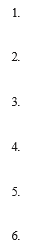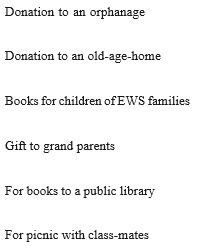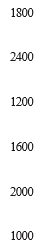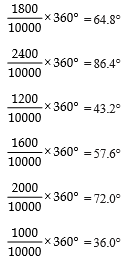Total 10,000 360°

The required pie-chart is as given below :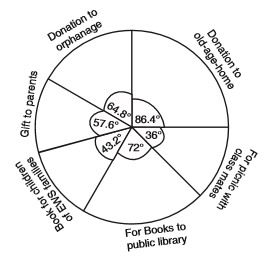(b) Statistics

(c)(i) gainful use of prize money
(ii) charity
(iii) caring for others

Question 2. 90 schools participated in a plantation programme. Each school planted 100 plants. After one month the number of plants that survived were recorded as :

 95 67 2E 32 65 69 33 98 96 16 42 32 3E 42 40 69 95 92 16 83 75 S3 85 37 65 63 42 89 65 73 31 49 64 76 S3 92 93, 68 52 79 81 59 82 75 82 86 90 44 62 31 3E 42 39 S3 87 56 5E 23 35 S3 85 30 68 69 S3 36 43 45 S3 75 66 S3 92 75 89 66 91 SS 89 53 42 93, 69 90 55 66 52 S3 34 36

(a) Present the data in a frequency table using classes 20-29, 30-39, ..... 90-99.
(b) From the table find the number of schools where 50% or more plants survived?
(c) Which mathematical concept is used in the above problem?
(d) By taking part in the plantation programme which value is depicted by the participating schools?
Sol.
(a) The required frequency table is given as: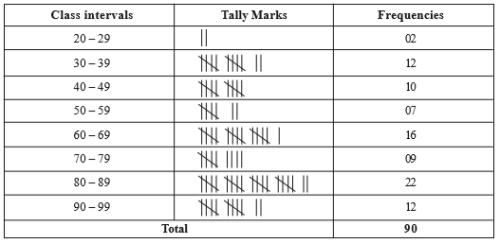(b) Total number of plants planted by each school = 100
∴ 50% of 100 = 50
⇒ Number of schools where 50% or more plants survived = 07 + 16 + 09 + 22 + 12 = 66
(c) Statistics
(d) Betterment of environment.

Question 3. 40 students of class IX contributed different amounts to Prime Minister’s Relief Fund. Vinod and Preeti were assigned to determine the mean amount of the class. Vinod comes out with his answer as 160 but Preeti found that the value of 165 was wrongly copied as 125 by Vinod and she gave the correct answer.

(a) Find the correct mean.
(b) Which concept of mathematics is used in the above problem?
(c) Which values are depicted by Preeti and other students of class IX?
Sol.
(a) Total number of students = 40
⇒ Number of observations = 40
∵ Mean calculated by Vinod = 160
∵ Total of 40 observations = 40 × 160 = 6400
But the observation 165 is recorded as 125
∴ Actual total of the 40 observations = (6400 – 125) + 165 = 6440
⇒ Correct mean calculated by Preeti = (6440/40)= 161
Thus, the correct mean is 161.

(b) Statistics

(c)(i) Accuracy
(ii) Patriotism

Question 4. Mrs. Prabha is a mathematics teacher in a school. She motivated 60 students from 2 sections of class IX to appear in proficiency-test in mathematics. The marks obtained by the students are as :

 57 31 46 74 6S 42 54 14 61 33 48 37 26 8 64 93 72 53 59 38 16 88 75 56 46 66 45 61 54 27 27 44 63 58 43 31 61 67 36 49 50 76 38 47 55 77 62 53 40 71 60 58 45 42 34 46 40 59 42 29
(a) Construct a group frequency distribution of the above data using classes 0 – 9, 10 – 19, etc. ...
(b) Find the number of students who secured more than 49 marks.
(c) Which mathematical concept is used in the above problem?
(d) By encouraging students of class IX in proficiency-test, which skill is depicted by Mrs. Prabha?
Sol. (a)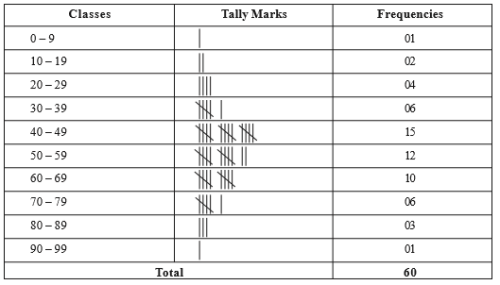(b) From the above frequency-table, we find that :
Number of students securing more than 49 marks = 12 + 10 + 6 + 3 + 1 =32
(c) Statistics
(d) Group inspiration.

Question 5. 30 students from senior classes of a school plan to donate blood in a blood donation camp.
Their blood groups are recorded as follows :

 B, A, o, o, AB, o, A, 0 B, A, B, A, o, o, A, AB o, A, A, o, o, AB, B, A o, B, A, o, B, 0

(a) Represent the given data in the form of a frequency distribution table.
(b) Which is the most common blood group?
(c) Which mathematical concept is used in the above problem?
(d) By donating blood, which value is depicted by the students of senior classes?
Sol. (a)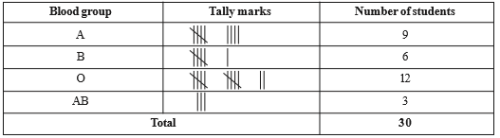(b) The most common blood group is ‘O’.

(c) Statistics

(d) Sacrificial act.

The document Value-Based Questions: Statistics Notes | Study Mathematics (Maths) Class 9 - Class 9 is a part of the Class 9 Course Mathematics (Maths) Class 9.
All you need of Class 9 at this link: Class 9

## Mathematics (Maths) Class 9

84 videos|352 docs|109 tests
 Use Code STAYHOME200 and get INR 200 additional OFF

## Mathematics (Maths) Class 9

84 videos|352 docs|109 tests

Track your progress, build streaks, highlight & save important lessons and more!

,

,

,

,

,

,

,

,

,

,

,

,

,

,

,

,

,

,

,

,

,

;